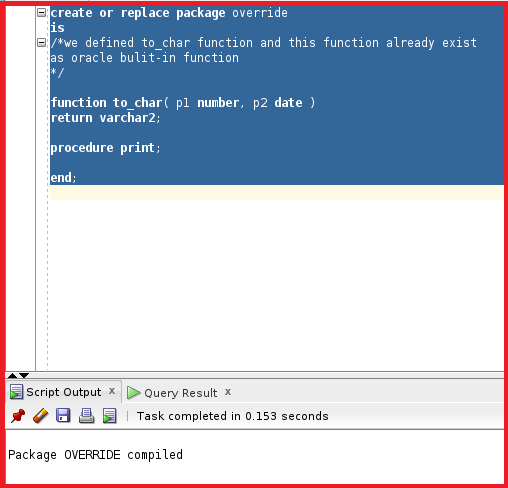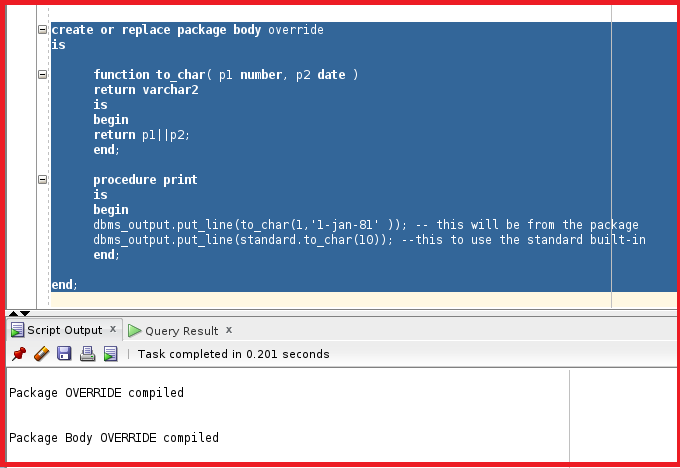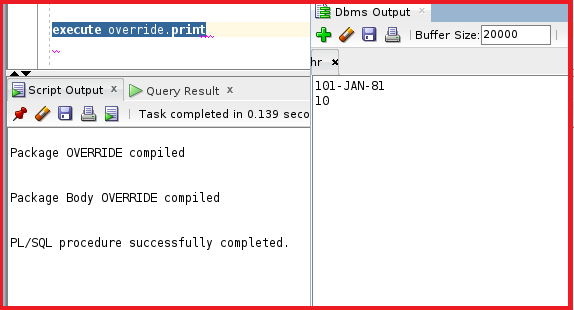```create or replace package override
is
function to_char( p1 number, p2 date )
return varchar2;
procedure print;
end;
```

So, the package contains one function and one procedure. The function takes the number and date as input and returns varchar2. The to_char is oracle standard and priority goes to this function. Let’s try to execute this package.The package specification is successfully compiled. We have two subprograms to_char and print. Let’s see the package body.

```create or replace package body override
is

function to_char( p1 number, p2 date )
return varchar2
is
begin
return p1||p2;
end;

procedure print
is
begin
dbms_output.put_line(to_char(1,'1-jan-81' )); -- this will be from the package
dbms_output.put_line(standard.to_char(10)); --this to use the standard built-in
end;

end;
```

The function will take two parameters as input p1 as number and p2 as date which will return by concatenating p1 and p2. The procedure print will have two to_Char function calls which contain one standard function and one is user-defined function. The user-defined function is created by us which takes two values as input 1 and 1-jan-81 and this is from the package and returns the concatenated value of these two parameters. The standard function takes only value 10 as the input and to call this standard package we have to use “standard. function_name”. Let’s now try to compile this package body.The package specification and package body are now compiled. Let’s now try to test this package by executing the package print.

execute override.print;So, from the DBMS output, we can see that the DBMS output is 101-jan-81 which is concatenated value. The second value is straight away from the standard function. This is the process of how we use the standard package.

In the next article, I am going to discuss Forward Declaration in Oracle with Examples. Here, in this article, I try to explain Overloading Standard Package in Oracle with Examples. I hope you enjoy this Overloading Standard Package in the Oracle article.